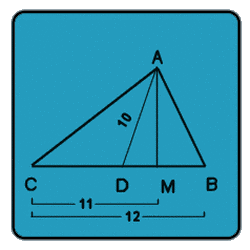All about flooble | fun stuff | Get a free chatterbox | Free JavaScript | Avatarsperplexus dot infoThe Unknown Side (Posted on 2002-07-01)ABC is a triangle:
• Angle A < 90.
• D is a point on BC such that BD = DC.
• M is a point on BC such that AM is perpendicular to BC.If
• AD = 10,
• BC = 12 and
• MC = 11
find the length of AC.
•  See The Solution Submitted by Dulanjana Rating: 2.9091 (11 votes)Comments: ( Back to comment list | You must be logged in to post comments.)Puzzle Resolution| Comment 14 of 15 |(In reply to answer by K Sengupta)

MB = BC-MC = 12-11 = 1
BC = 12, and so: BD = DC =6
Thus, DM = DB - MB = 6-1=5

Accordingly, AM = V(10^2 - 5^2) = V(75)

But MC = 11, and so:
AC = sqrt(AM^2 + MC^2)
= sqrt(75+121)
= sqrt(196)

 Posted by K Sengupta on 2007-06-15 12:21:11Please log in:

 Search: Search body:
Forums (0)